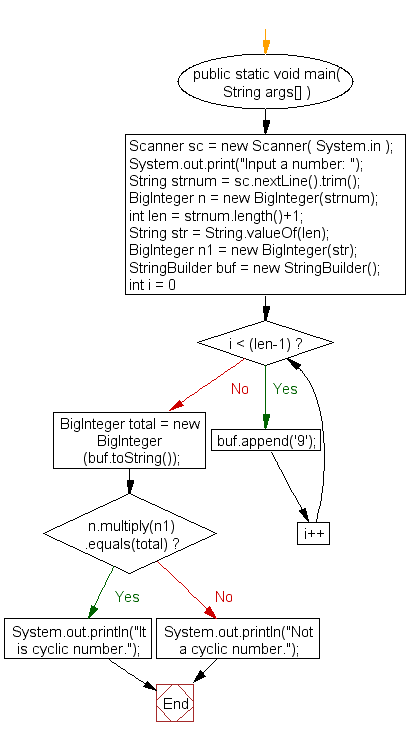﻿ Java exercises: Check a number is a cyclic or not - w3resource# Java Exercises: Check a number is a cyclic or not

## Java Numbers: Exercise-19 with Solution

Write a Java program to check a number is a cyclic or not.

A cyclic number is an integer in which cyclic permutations of the digits are successive multiples of the number. The most widely known is 142857:
142857 × 1 = 142857
142857 × 2 = 285714
142857 × 3 = 428571
142857 × 4 = 571428
142857 × 5 = 714285
142857 × 6 = 857142
Input Data:
Input a number: 142857

Pictorial Presentation:Sample Solution:

Java Code:

``````import java.util.Scanner;
import java.math.BigInteger;
public class Example19 {

public static void main( String args[] ){
Scanner sc = new Scanner( System.in );
System.out.print("Input a number: ");
String strnum = sc.nextLine().trim();
BigInteger n = new BigInteger(strnum);
int len = strnum.length()+1;
String str = String.valueOf(len);
BigInteger n1 = new BigInteger(str);
StringBuilder buf = new StringBuilder();
for(int i = 0 ; i < (len-1); i++) {
buf.append('9');
}
BigInteger total = new BigInteger(buf.toString());
if(n.multiply(n1).equals(total)) {
System.out.println("It is a cyclic number.");
}
else {
System.out.println("Not a cyclic number.");
}
}
}
```
```

Sample Output:

```Input a number: 142857
It is a cyclic number.
```

Flowchart:Java Code Editor:

What is the difficulty level of this exercise?

﻿

## Java: Tips of the Day

Array vs ArrayLists:

The main difference between these two is that an Array is of fixed size so once you have created an Array you cannot change it but the ArrayList is not of fixed size. You can create instances of ArrayLists without specifying its size. So if you create such instances of an ArrayList without specifying its size Java will create an instance of an ArrayList of default size.

Once an ArrayList is full it re-sizes itself. In fact, an ArrayList is internally supported by an array. So when an ArrayList is resized it will slow down its performance a bit as the contents of the old Array must be copied to a new Array.

At the same time, it's compulsory to specify the size of an Array directly or indirectly while creating it. And also Arrays can store both primitives and objects while ArrayLists only can store objects.

Ref: https://bit.ly/3o8L2KH